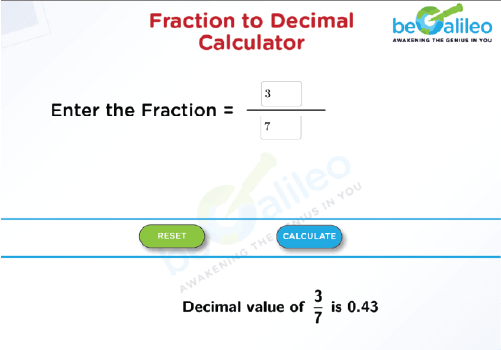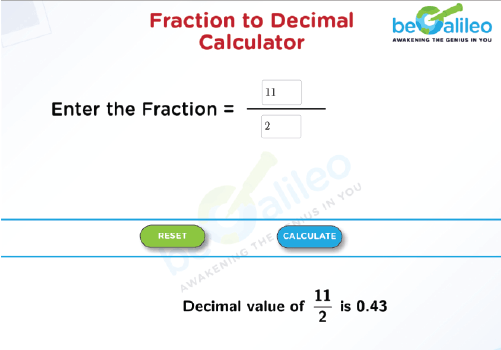>### Master Math with 1:1 Online Classes. Learn from Experts!# Fraction to Decimal Calculator

Decimals and fractions are two different methods to express numbers. While decimals are used to represent numbers more precisely, fractions are used to represent pieces of a whole. A Fraction to Decimal Calculator is a tool or program designed to automate the process of converting fractions into decimal numbers. Instead of manually performing the division required to convert fractions to decimals, you can use this calculator to obtain the decimal representation quickly and accurately.

## How to use the Fraction to Decimal Calculator?

1. Input :
Begin by entering the numerator (top number) and denominator (bottom number) of the fraction you wish to convert.
2. Calculation :
Our calculator automatically performs the division, converting your fraction into a decimal value.
3. Output :
The result is then displayed as a decimal number.
4. Reset Option :
If needed, you can use the reset button to clear all entered numbers, allowing you to correct input errors or start a new calculation.

By following these steps, you can efficiently utilize our Fraction to Decimal Calculator for your decimal conversion needs.

## How to convert Fraction to Decimal?

• To convert a fraction to a decimal, you can use long division.

Here are the steps for converting a fraction to a decimal using long division:

1. Divide the numerator (the top number) by the denominator (the bottom number).
2. Perform the division, keeping track of the quotient and any remainders. Continue the division until you reach your desired level of decimal precision or until the remainder becomes zero, indicating that the division is complete.
3. The result you obtain from the division is the decimal representation of the fraction.

Example :
Let's go through an example using the fraction \frac{3}{4}:
\frac{3}{4}\ \Longrightarrow \ 3 \divide 4

Setup : Place the dividend (3.00) inside the division symbol and the divisor (4) outside.

First Division : Divide the leftmost digit of the dividend (3) by the divisor (4), yielding a result of 0 with a decimal point.

Bring Down : Move to the next digit in the dividend (0).

Second Division : Divide 30 by 4, resulting in 0.7 with a remainder of 2.

Bring Down Again : Include the extra zero from the dividend.

Third Division : Divide 20 by 4.

Result Refinement : The outcome is 0.75 with no remainder.

Repeat : Continue this process until you achieve the desired level of precision or until the division terminates. In this case, the division terminates because a finite decimal result is reached.

• Conversion of denominator into multiple of 10.

The process of converting a fraction to a decimal number is simple when the denominator of the fraction is a multiple of 10, 100, 1000, 10000, etc. Depending on how many zeros there are in the denominator, the decimal point is first written down and then moved to the left, starting with the last digit of the number.

Example :
Convert the fraction \frac{7513}{100}to a decimal.
\frac{7513}{100}=75.13

## Solved examples

### Q1. Convert the fraction \frac{3}{7}to a decimal.

Solution :
\frac{3}{7}=0.428571### Q2. Convert the mixed fraction 5\frac{1}{2}to a decimal.

Solution :
5\frac{1}{2} = \frac{11}{2} = 5.5